Some months ago, I wrote about being busy writing papers both to the ICEM and EPNC conferences, the latter of which is being organized by our research group in Helsinki next month. Now, I’m happy to announce both were accepted with minor revisions! Since I’m not going to submit the EPNC paper to any journal later, you can already find the full pre-print version on my Researchgate page. As you can see, it’s about reluctance networks.

### Reluctance networks basics

Reluctance networks are one version of the so-called lumped-parameter models for analysing electrical machines, or any other device where the behaviour of magnetic fields is of interest. They are based on at least some a-priori assumptions about how the flux will flow inside the machine. In other words, they require at least one degree more of user expertise or insight compared to the finite element method.

For instance, the flux in the tooth of a machine can be expected to travel mostly in the direction of the tooth, either towards to air-gap or away from it. Therefore, we could probably model the tooth with a single reluctance element.

Inside the element – often also called flux tube – the flux can only travel between the two ends of the element, i.e. not leak through its walls. That’s just a limiting and simplifying assumption that we make. Moreover, we assume that the both of the reluctance element ends are equipotential surfaces for the so-called magnetic scalar potential.

Mathematically speaking, we assume that the line integral of the magnetic field strength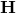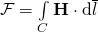is a constant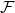, regardless of what path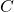you take between the two ends of the element.

Thiswe call the drop in the magnetomotive force, or mmf, over the element. Moreover, we relate the total flux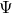going through the element, and the mmf drop, with the quantity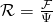.

This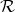is what we call the reluctance, i.e. the value assigned to the reluctance element.

A clarifying example is probably necessary. Let’s consider an electrical circuit, and its simplest component of all: the resistor. For a resistor, we know that the voltage over the resistor and the current going through it are proportional to each other, with their ratio equal to the resistance of the resistor.

The resistor is pretty much fully equivalent to a reluctance element. Only instead of current, we have flux. And instead of voltage drop, we have mmf drop. And finally, the term reluctance is to the reluctance element what resistance is to a resistor.

Nonsurprisingly, the reluctance network is then formed by connecting several reluctance elements to each other. And since it is equivalent to an electrical network, it handily satisfies the conservation laws we have with magnetic fields.

What I mean that like no current disappears in an electric circuit, no flux will vanish in a reluctance network either. Likewise, the sum of mmf drops in any closed loop in the network will equal the sum of mmf sources (more of them later) in that loop. Exactly like what happens with voltages in an electric circuit, you see.

### Uses

The main benefit of a reluctance network is that the number of unknowns, be it the fluxes or mmfs, can be very low compared to e.g. FEM model of the same device. Thus, they can be very fast to solve, and still offer comparable accuracy.

Indeed, the figure below shows some results from the conference paper. The reference solution was calculated with the commercial FEM software Comsol, taking something like two hours. By contrast, the reluctance network solution was obtained in something like 40 seconds.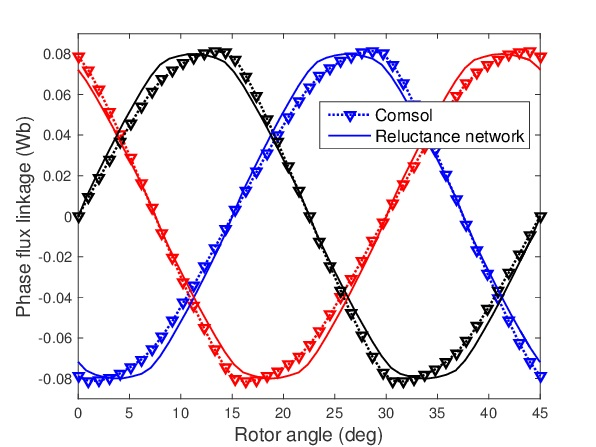The phase flux linkages of an axial flux permanent magnet machine, calculated with a reluctance network and a commercial FEM software.

That’s a quite nice speed-up, isn’t it?

In the future, I’ll tell you how to actually solve the network with a computer, and more about my paper in particular. However, the very next post in the series is going to be a word of caution, instead.

Need help with electric motor design or design software? Let's get in touch - satisfaction guaranteed!Reluctance networks 101